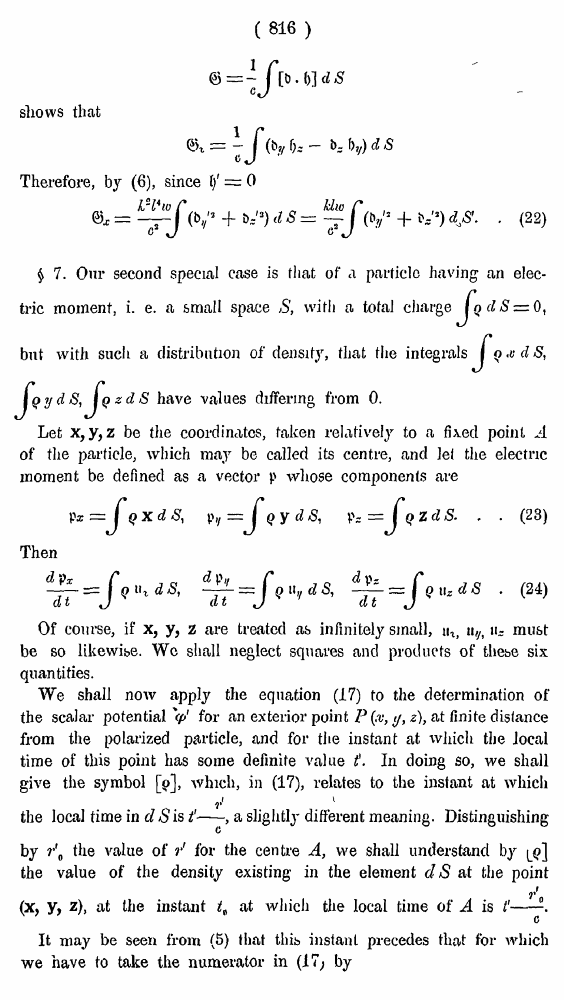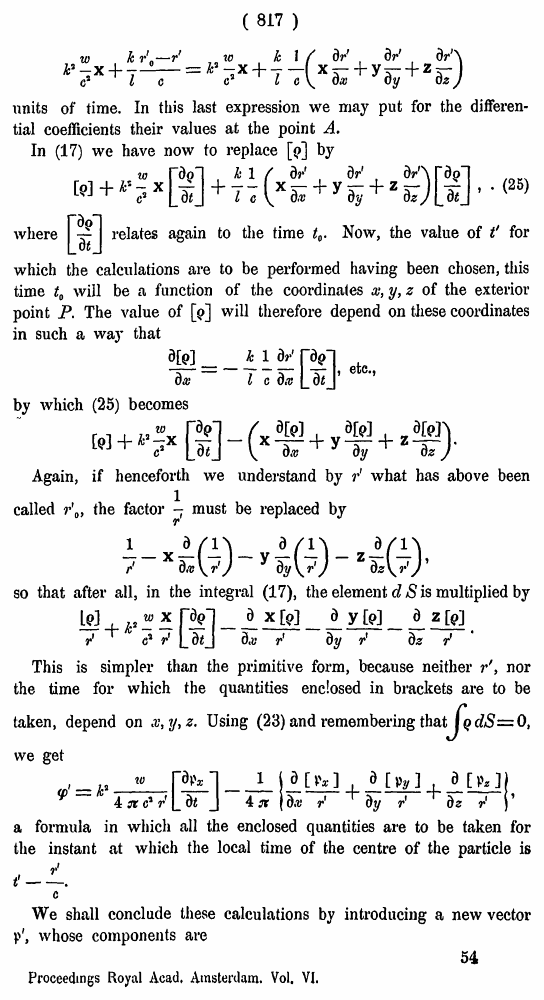# H. A. Lorentz. Electromagnetic phenomena in a system moving with any velocity smaller than that of light. // Proceedings Royal Acad., Amsterdam. Vol. VI., 1904

В начало   Другие форматы   <<<     Страница 816   >>>809  810  811  812  813  814  815  816 817  818  819  820  821  822  823  824  825  826  827  828  829  830  831 ( 816 ) shows that Therefore, by (6), since 1/ = 0 • (22) \$ 7. Our second special case is that of a particle having an electric moment, i. e. a small space S, with a total charge, but with such a distribution of density, that the integrals have values differing from 0. Let x,y, z be the coordinates, taken relatively to a fixed point of the particle, which may be called its centre, and lei the electric moment be defined as a vector p whose components are . . (23) Then • (24) Of course, if x, y, z are treated as infinitely small, ua, u,h «- must be so likewise. We shall neglect squares and products of thebe six quantities. We shall now apply the equation (17) to the determination of the scalar potential V for an exterior point P(x, y, z), at finite distance from the polarized particle, and for the instant at which the Jocal time of this point has some definite value \$. In doing so, we shall give the symbol , which, in (17), relates to the instant at which I the local time in d S isa slightly different meaning. Distinguishing by r\ the value of r' for the centre A, we shall understand by the value of the density existing in the element dS at the point (X, y, z), at the instant tt at which the local time of A is It may be seen from (5) that this instant precedes that tor which we have to take the numerator in (17; by (817) units of time. In this last expression we may put for the differential coefficients their values at the point A. In (17) we have now to replace [j>] by . • (25) whererelates again to the time t0. Now, the value of t' for which the calculations are to be performed having been chosen, this time t0 will be a function of the coordinates x, y, z of the exterior point P. The value of  will therefore depend on these coordinates in such a way that etc., by which (25) becomes Again, if henceforth we understand by r‘ what has above been called r\, the factoimust be replaced by so that after all, in the integral (17), the element cl S is multiplied by This is simpler than the primitive form, because neither r', nor the time for which the quantities enclosed in brackets are to be taken, depend on x, y, z. Using (23) and remembering thai we get a formula in which all the enclosed quantities are to be taken for the instant at which the local time of the centre of the particle is We shall conclude these calculations by introducing a new vector V', whose components are 54 Proceedings Royal Acad, Amsterdam. Vol. VI.